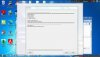# WindowsDoAdd Execution Error

#### Proeerikactive

##### Member
Hi!
Today I was playing the game i made, called JeweLevel.
I finished a level of Normal free-play mode when suddenly, during the transition to the next level,The strange thing is that the transition of this mode is the same as other free play modes Classic and Timed except before it, you can either continue or save and quit. The other 2 had nothing wrong.
Also, this is a finished game and when i published it, there was nothing wrong with Normal, and i don't remember doing anything to the level start code during recent updates.
If this helps, here is the create event of ob_brain
GML:
``````if instance_exists(ob_preserve) = false
{
globalvar busy, bx, by, supermatch, last_jewel;
busy = true;
bx = 0;
by = 0;
supermatch = 0;
global.points = 0;
global.combo = 1;
global.play = 1;
if global.level > 0
{
if global.mode < 4
{
global.target = 1000;
global.level = 1;
}
global.target_add = 2000;
if global.mode < 3
{
global.level_multi = 2;
}
else
{
global.level_multi = 1;
}
}
instance_create(x,y,ob_preserve);
global.lost = 0;
global.move_made = 0;
if (global.music = 1)
{
alarm = 5;
if (global.mode = 1) or (global.mode = 3) or (global.adv_mode = 1)
{
audio_group_load( audiogroup_normal);
}
else if (global.mode = 2) or (global.adv_mode = 2)
{
audio_group_load( audiogroup_timed);
}
else
{
audio_group_load(audiogroup_bonus);
}
}
if (global.mode = 4) and (global.level = 1)
{
instr = instance_create(576,352,ob_part_instruct);
(instr).image_index = 0;
}
if (global.mode = 4) and (global.level = 6)
{
instr = instance_create(576,352,ob_part_instruct);
(instr).image_index = 1;
}
if (global.mode = 4) and (global.level = 10)
{
instr = instance_create(576,352,ob_part_instruct);
(instr).image_index = 2;
}
if (global.mode = 4) and (global.level = 16)
{
instr = instance_create(576,352,ob_part_instruct);
(instr).image_index = 4;
}
if (global.mode = 4) and (global.level = 31)
{
instr = instance_create(576,352,ob_part_instruct);
(instr).image_index = 5;
}
if (global.mode = 4) and (global.level = 46)
{
instr = instance_create(576,352,ob_part_instruct);
(instr).image_index = 6;
}
if (global.mode = 4) and (global.level = 61)
{
instr = instance_create(576,352,ob_part_instruct);
(instr).image_index = 7;
}
if (global.mode = 4) and (global.level = 76)
{
instr = instance_create(576,352,ob_part_instruct);
(instr).image_index = 8;
}
if (global.mode = 4) and (global.level = 106)
{
instr = instance_create(576,352,ob_part_instruct);
(instr).image_index = 9;
}
if (global.mode = 4) and (global.level = 121)
{
instr = instance_create(576,352,ob_part_instruct);
(instr).image_index = 10;
}
if (global.mode = 4) and (global.level = 146)
{
instr = instance_create(576,352,ob_part_instruct);
(instr).image_index = 11;
}
if (global.mode = 4)
{
if (global.level < 16)
background_index = 0;
else if (global.level < 31)
background_index = 1;
else if (global.level < 46)
background_index = 2;
else if (global.level < 61)
background_index = 3;
else if (global.level < 76)
background_index = 4;
else if (global.level < 91)
background_index = 5;
else if (global.level < 106)
background_index = 6;
else if (global.level < 121)
background_index = 7;
else if (global.level < 136)
background_index = 8;
else background_index = 9;
}
if (global.adv_mode = 5)
instance_create(144,576,ob_req_jewel);
if (global.multigem = 1)
instance_create(144,576,ob_bar);
}
else
{
globalvar busy, bx, by, supermatch, last_jewel;
busy = true;
bx = 0;
by = 0;
supermatch = 0;
global.play = 1;
global.target += global.target_add;
global.target_add += 1000;
if global.mode = 1
{
global.level_multi += 1;
}
else
{
global.level_multi += 0.5;
}
global.level += 1;
background_index = (global.level - 1) mod 10;
global.move_made = 0;
global.combo = 1;
}
delay = 5;
checking = 0;
global.multiplier = 1;
if global.level = -1
background_index = 11
background_xscale = room_width / background_width;
background_yscale = room_height / background_height;
instance_create(144,320,ob_block_decorate)
paused = 0
global.combo_high = 0
global.moves_used = 0
global.move_best = 0
global.gem_destroy = 0
global.power_create = 0
global.oldscore = global.points
global.basic = 1``````
Also, error in line -1?!